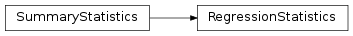mvpa2.clfs.transerror.RegressionStatistics¶class mvpa2.clfs.transerror.RegressionStatistics(**kwargs)

Class to contain information and display on regression results.

Attributes

 error sets stats summaries Return a list of separate summaries per each stored set

Methods

 add(targets, predictions[, estimates]) Add new results to the set of known results as_string([short, header, summary, description]) ‘Pretty print’ the statistics compute() Actually compute the confusion matrix based on all the sets plot([plot, plot_stats, splot]) Provide presentation of regression performance in image reset() Cleans summary – all data/sets are wiped out

Initialize RegressionStatistics

Parameters: targets Optional set of targets predictions Optional set of predictions

Attributes

 error sets stats summaries Return a list of separate summaries per each stored set

Methods

 add(targets, predictions[, estimates]) Add new results to the set of known results as_string([short, header, summary, description]) ‘Pretty print’ the statistics compute() Actually compute the confusion matrix based on all the sets plot([plot, plot_stats, splot]) Provide presentation of regression performance in image reset() Cleans summary – all data/sets are wiped out